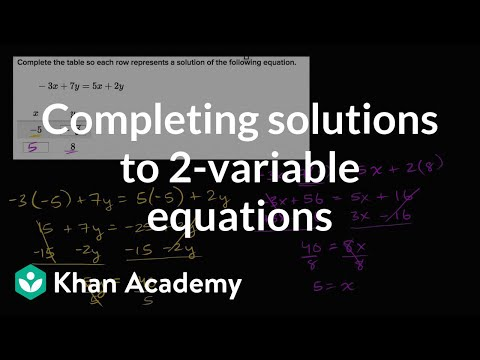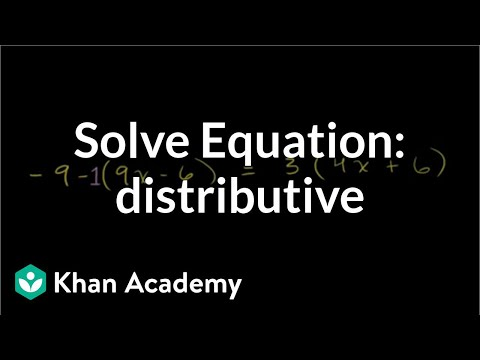COVID-19 presents an unprecedented challenge for teachers, students, and parents. Explore resources from IBM and partners here.
Video

# Equation with variables on both sides: fractions (Full video)

Khan Academy

## Supporting grades: 8

Description: Sal solves the equation (3/4)x + 2 = (3/8)x - 4 using the distributive property. We have the equation 3/4x plus 2 is equal to 3/8x minus 4. So what I'm going to do, right from the start of this video, is to multiply both sides of this equation by some number so I can get rid of the fractions. I'm going to multiply 8 times both sides of this equation.

You must log inorsign upif you want to:*

*Teacher Advisor is 100% free.

### Other videos you might be interested in### Completing solutions to 2-variable equations (Full video)

#### Khan Academy